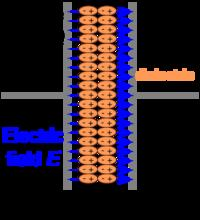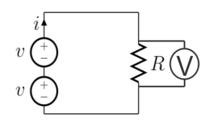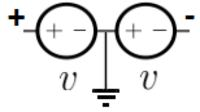# Negative voltage - what is it? what is it for?

Status
Not open for further replies.

#### Vermes

What is negative voltage? To answer this question, we need to know what is electric current and positive voltage. The answer is quite obvious for most of us, but I'll write it down just for the record.

The electrical current is an ordered flow of electrical charge (electrons) through an electrical conductor. Electrical currents flow when there is voltage present across a conductor.

To have an electrical flow, you have to have some sort of tension, something which will cause the electrons flow. This tension is called voltage – you can have positive and negative voltage which precisely refers to the direction of the flow. Please look at the picture below:What you can see here is some sort of power supply, for now (and for the simplicity of this sheet) let's assume that it is a battery (a 9V battery) and a resistor with for example 100Ohm of resistance. From the Ohm's law we learn that resistance is proportional to Voltage divided by Current R=U/I, therefore, if there was no resistor, it would look like that: 0=U/I and then the current would go to infinity (create a short circuit) and damage the power supply (if it hadn't got any protection). This was out of topic, lets get back to voltage.
Imagine a glass of water with a pipe on its bottom. The glass is filled with water which flows through it. Why is it flowing? Because we have gravity. The same situation is when we are talking about electrical current - it flows because we have electrical tension – Voltage. Unlike gravity, we know precisely where does this tension come from. Please look at a very simple example – a capacitor:In the above picture, we have two plates separated by a dielectric. An electric field is created between the plates – because of plus and minus poles, the loads “want” to connect. You can imagine it to be like a magnet. For our need, let's assume that this dielectric is perfect and the capacitor, battery doesn't discharge itself (in the real world it discharges a little through the filed, but it's not important here in this example).
We have a tension here. Now, if we connect the plates by another way (as on the first picture) we use this tension to get a electrical flow. We know the direction of the flow, and it's from plus to minus (it was adopted like this – looking at physics, the electric flow goes from – to +) we have a positive tension. Please look at the picture below:We added a voltmeter on the resistor to measure the voltage. Due to the fact that we used a 9V battery, the voltage will be 9V. To enlarge the voltage, you could connect a next battery. But now – should it be connected in parallel or in series. If you don't see it in electrics, imagine the glass which was mentioned previously. If we connect a next glass, it doesn't enlarge the water column, therefore, if it doesn't enlarge the tension – it enlarges the capacity. If you want to enlarge the tension (voltage), you need to connect it in series.Due to the fact that the batteries have 9V, the voltmeter will show 18V, if the voltmeter will be connected like the batteries (- in the start and + in the end) → in accordance with the current flow. The voltage sing depends on the voltmeter connection, if it's connected along the current flow, the voltage is positive, but if you connect it in the opposite direction, the sign will be negative.
Okay. I hope you all understand now everything about the positive voltage, now let's get back to the main topic of this article → negative voltage.
The negative voltage means that the tension is in the other direction. The easiest way to get a negative voltage is to create a circuit like this presented below:If the supplies are 9V, on the left side we have +9V, and on the right -9V. GND is in the middle of the batteries.

Where do we need negative voltage?
1. If you would like to create for example a sin wave, an easy way to do this is to use a RC filter and an operational amplifier powered by positive and negative voltage (a basic supply is +15V -15V),
2. Another example is to power a multiplexer – many devices like this use positive and negative voltage,
3. Some sensors use negative voltage – for example load and torque sensors,
4. PMOS switches
5. RS232 communication (you can use IC like MAX232 which does it by a charge pump, therefore, you don't need to create your own negative supply)

Status
Not open for further replies.